# Converting Fractions to Mixed Numbers

## Learn About Converting Fractions to Mixed Numbers With Example Problems And Interactive Exercises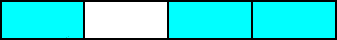You may recall the example below from a previous lesson.

Example 1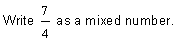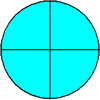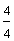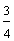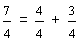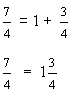In example 1, we used circles to help us solve the problem. Now look at the next example.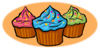Example 2: At a birthday party, there are 19 cupcakes to be shared equally among 11 guests. What part of the cupcakes will each guest get?

Analysis: We need to divide 19 cupcakes by 11 equal parts. It would be time-consuming to use circles or other shapes to help us solve this problem. Therefore, we need an arithmetic method.

Step 1: Look at the fraction nineteen-elevenths below. Recall that the fraction bar means to divide the numerator by the denominator. This is shown in step 2.

Step 2:Step 3:Solution: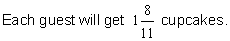Example 3:Step 1:Step 2: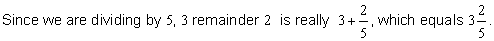AnswerAnalysis: We need to divide 37 into 10 equal parts.

Step 1:Step 2:Answer:Example 5:Analysis: We need to divide 37 into 13 equal parts.

Step 1: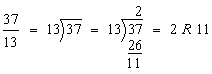Step 2: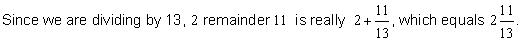Answer:In each of the examples above, we converted a fraction to a mixed number through long division of its numerator and denominator. Look at example 6 below. What is wrong with this problem?

Example 6:Analysis: In the fraction seven-eighths, the numerator is less than the denominator. Therefore, seven-eighths is a proper fraction less than 1. We know from a previous lesson that a mixed number is greater than 1.

Answer: Seven-eighths cannot be written as a mixed number because it is a proper fraction.

Example 7: Can these fractions be written as mixed numbers? Explain why or why not.Analysis: In each fraction above, the numerator is equal to the denominator. Therefore, each of these fractions is an improper fraction equal to 1. But a mixed number is greater than 1.

Answer: These fractions cannot be written as mixed numbers since each is an improper fraction equal to 1.

After reading examples 6 and 7, you may be wondering: Which types of fractions can be written as mixed numbers? To answer this question, let's review an important chart from a previous lesson.

Comparison of numerator and denominator: If the numerator <  denominator, then the fraction < 1

Example:Type of Fraction: proper fraction

Write As: proper fraction

Comparison of numerator and denominator: If the numerator = denominator, then the fraction = 1.

Example: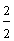Type of Fraction: improper fraction

Write As: whole number

Comparison of numerator and denominator: If the numerator > denominator, then the fraction > 1.

Example: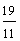Type of Fraction: improper fraction

Write As: mixed number

The answer to the question is: Only an improper fraction greater than 1 can be written to a mixed number.

Summary: We can convert an improper fraction greater than one to a mixed number through long division of its numerator and denominator.

### Exercises

In Exercises 1 through 5, click once in an ANSWER BOX and type in your answer; then click ENTER. After you click ENTER, a message will appear in the RESULTS BOX to indicate whether your answer is correct or incorrect. To start over, click CLEAR. Note: To write the mixed number four and two-thirds, enter 4, a space, and then 2/3 into the form.

 1. Write eleven-fifths as a mixed number.ANSWER BOX:   RESULTS BOX:
 2. Write eleven-fourths as a mixed number.ANSWER BOX:   RESULTS BOX:
 3. Write thirteen-ninths as a mixed number.ANSWER BOX:   RESULTS BOX:
 4. On field day, there are 23 pies to share equally among 7 classes. What part of the pies will each class get?ANSWER BOX:   RESULTS BOX:
 5. A teacher gives her class a spelling test worth 35 points. If there are 8 words graded equally, then how many points is each word worth?ANSWER BOX:   RESULTS BOX:
 Lessons on Fractions 1. Introduction to Fractions 2. Classifying Fractions 3. Equivalent Fractions 4. Simplifying Fractions 5. Comparing Fractions 6. Ordering Fractions 7. Converting Fractions to Mixed Numbers 8. Converting Mixed Numbers to Fractions 9. Practice Exercises 10. Challenge Exercises 11. Solutions